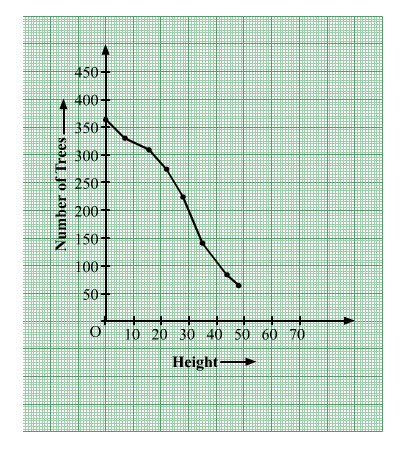# The following table gives the height of trees:

Question:

The following table gives the height of trees: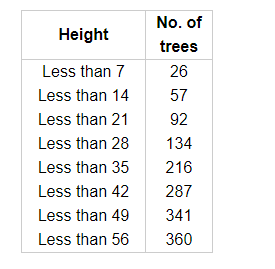Draw 'less than' ogive and 'more than' ogive.

Solution:

Consider the following table.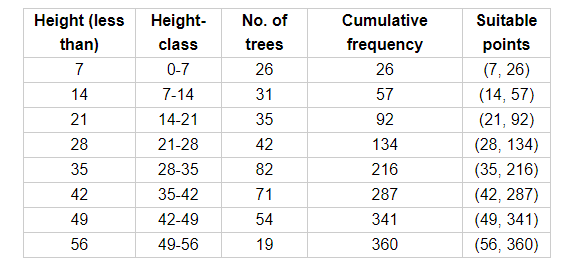Now, draw the less than ogive using suitable points.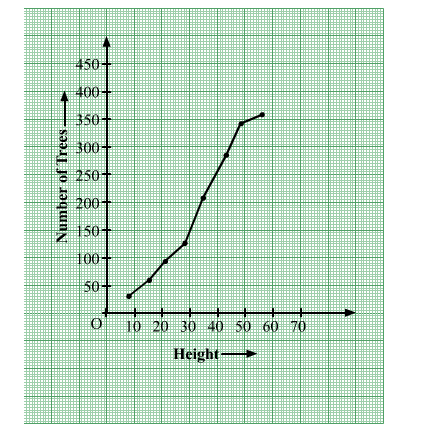Now, prepare the cumulative frequency table for more than series.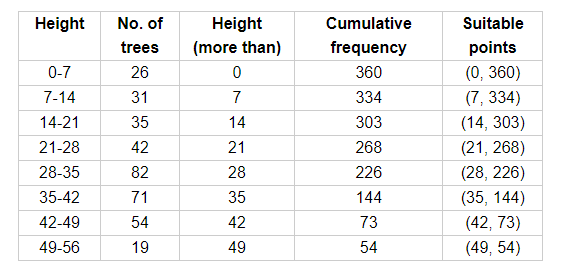Now, draw the more than ogive using suitable points.